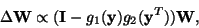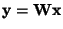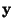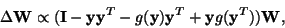Next: Algorithms for maximum likelihood Up: Algorithms for ICA Previous: Jutten-Hérault algorithm

## Non-linear decorrelation algorithms

Further algorithms for canceling non-linear cross-correlations were introduced independently in [34,33,30] and [91,28]. Compared to the Jutten-Hérault algorithm, these algorithms reduce the computational overhead by avoiding any matrix inversions, and improve its stability. For example, the following algorithm was given in [34,33]:(34)

where, the non-linearities g1(.) and g2(.) are applied separately on every component of the vector, and the identity matrix could be replaced by any positive definite diagonal matrix. In [91,28], the following algorithm called the EASI algorithm was introduced:(35)

A principled way of choosing the non-linearities used in these learning rules is provided by the maximum likelihood (or infomax) approach as described in the next subsection.

Aapo Hyvarinen
1999-04-23